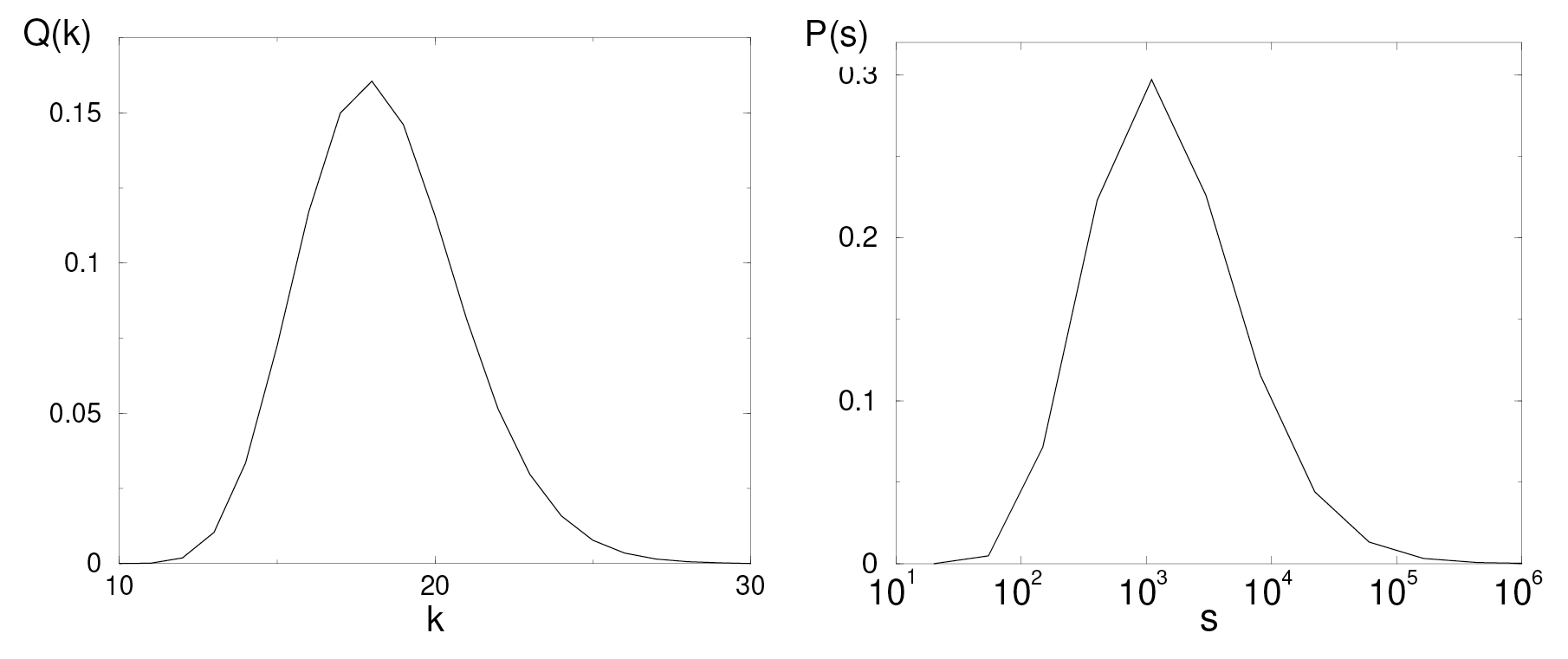##### May 4, 2020

One can’t help noticing that forecasts of the COVID-19 epidemic’s toll are inconsistent with each other, and a single forecast can change dramatically over a few days. Recent estimates for the U.S. epidemic death toll have ranged from tens of thousands to millions, with the current number around 64,000. Given these uncertainties, one might conclude that none of these estimates is trustworthy. More perniciously, the wildly disparate estimates might cause a reasonable person to doubt expertise and thus rely on sources that confirm her/his own personal bias.

Our purpose is to show that huge forecasting uncertainties are an integral feature of processes that exhibit exponential growth, an oft-misused term in the lay literature. Exponential growth refers to a time-dependent process in which the size of some quantity today is its size yesterday times a factor that is greater than 1. As an example, suppose you deposit $1 in a bank that pays 1 percent interest per day. How long would it take to become a millionaire? One day later, your account is worth$1.01; two days later $(1.01)2, three days later$(1.01)3, etc. After n days your account is worth $(1.01)n. Setting$(1.01)n equal to $1 million leads to n = ln 106 ln 1.01 ≈ 1388 days, or roughly 3.8 years. It’s a short wait to become a millionaire at this bank. If your bank is more generous and pays 2 percent daily interest, you would get$1 million in 697 days, or 1.9 years. With 5 percent interest, in 9.3 months; with 10 percent interest, just under five months. As an important corollary, if you initially deposited \$10 at 10 percent interest, your fortune would arise in four months. The initial value of the deposit hardly matters; it’s the exponential growth that’s crucial.

Let’s now turn to epidemic forecasting. Without any mitigation, each COVID-19 patient infects an additional 2.5 people, on average, in a single incubation period. This factor 2.5 is termed the reproductive number R0. Note that R0 = 2.5 corresponds to 250 percent interest, for which the entire planet would be infected after only 25 incubation periods. The goal of social distancing and related interventions is to reduce the reproductive number to below 1, after which the epidemic would stop. We estimate how many people would end up getting infected when the epidemic stops.

Suppose that public health authorities detect the epidemic when N people are infected, after which mitigation strategies are imposed to reduce R0 to less than 1. Because society is complex, it is not possible to reduce R0 instantaneously, but rather, it happens gradually. Thus our model: each successive day R0 is decreased by a random number r whose average value ⟨r⟩ is less than 1. (For simplicity of language, we use “days” rather than “incubation period” as the basic time unit.) The number of days k until R0 reaches 1 is determined by R0rk = 1 or k = ln 0.4 ln ⟨r⟩. Thus, if R0 decreases by a factor ⟨r⟩ = 0.95 daily, the epidemic is extinguished in 18 days.Figure 1. (a) The probability Q(k) that the epidemic lasts k days. (b) The probability P(s) that the epidemic has increased in size by a factor s from its initial size.

We simulate this mitigation strategy by starting with reproductive number R0 = 2.5 and choosing random numbers r1r2, r3, . . ., each of which is uniformly distributed between 0.9 and 1, so that ⟨r⟩ = 0.95. The reproductive number after k days of this mitigation is the product Rk = R0 r1r2, r3 . . . rk. We measure how long it takes until Rk is reduced to 1 and then perform this same measurement for 106 different choices of the set of random numbers r1r2, . . . , rk. As shown in figure 1(a), the probability Q(k) that the epidemic is extinguished in k days is peaked at roughly 18 days, as we expect. If one is lucky, that is, if more of the reduction factors ri are less than 0.95, the epidemic is extinguished in as little as 13 days. If one is unlucky (e.g., many of the ri are close to 1), the epidemic can last more than 25 days.  However, the probability of these extreme events is small and almost all instances of the epidemic last close to 18 days.

Here is the crucial point: at the end of the epidemic, the size of different instances of the outbreak can be different by several orders of magnitude, that is, by several factors of 10. As shown in figure 1(b), while the most likely epidemic size is s = 1,000N, there is an appreciable probability that the outbreak size can be as small as 100N or as large as 10,000N! This huge disparity in outbreak size is the salient point — tiny changes in how the epidemic is mitigated can lead to huge changes in the outbreak size.

More dramatically, consider a less effective mitigation in which the reproductive number is reduced, on average, by 0.975 rather than 0.95. Now the epidemic lasts between 30 and 45 days, with an average duration of 36 days. However, the epidemic size ranges between 500,000N and 50,000,000N, with an average value of 3,000,000N. Although the epidemic lasts twice as long, it is typically 3,000 times larger!

It bears emphasizing that this idealized model represents a dramatic oversimplification of realistic epidemic mitigation strategies. However, the model captures the huge uncertainties that arise in predicting the final outbreak size. This unpredictability is intrinsic to epidemic dynamics and not indicative of shortcomings in modeling. In short, forecasting ambiguity is unavoidable in exponential growth processes that underlie epidemics.

Sidney Redner
Santa Fe Institute

T-028 (Redner) PDF

Read more posts in the Transmission series, dedicated to sharing SFI insights on the coronavirus pandemic.

Listen to David Krakauer discuss this Transmission on episode 32 of our Complexity Podcast.